Trigonometry : Phase Shifts

Example Questions

Example Question #1 : Phase Shifts

Identify the phase shift of the following equation.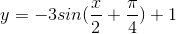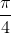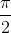Explanation:

If we use the standard form of a sine function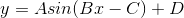the phase shift can be calculated by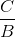.  Therefore, in our case, our phase shift is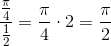Example Question #2 : Phase Shifts

Which of the following is equivalent to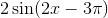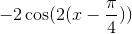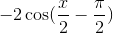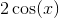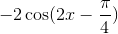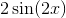Explanation:

The first zero can be found by plugging 3π/2 for x, and noting that it is a double period function, the zeros are every π/2, count three back and there is a zero at zero, going down.

A more succinct form for this answer is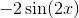but that was not one of the options, so a shifted cosine must be the answer.

The first positive peak is at π/4 at -1, so the cosine function will be shifted π/4 to the right and multiplied by -1. The period and amplitude still 2, so the answer becomes.

To check, plug in π/4 for x and it will come out to -2.

Example Question #3 : Phase Shifts

Which of the following is the correct definition of a phase shift?

The distance a function is shifted horizontally from the general position

A measure of the length of a function between vertical asymptotes

The distance a function is shifted vertically from the general position

The distance a function is shifted diagonally from the general position

The distance a function is shifted horizontally from the general position

Explanation:

Take the function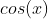for example.  The graph foris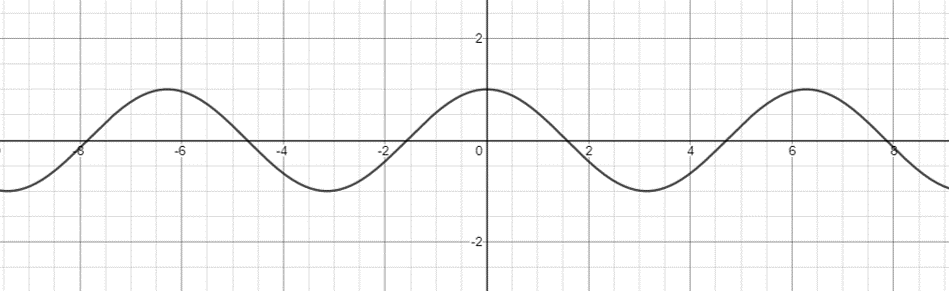If we were to change the function to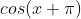, our phase shift is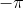.  This means we need to shift our entire graph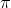units to the left.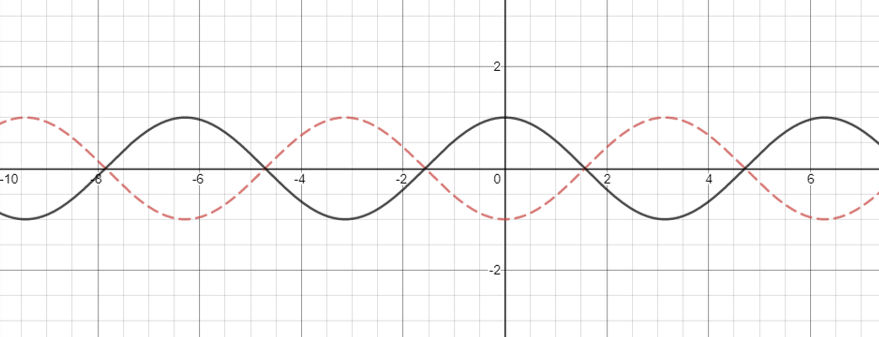Our new graphis the following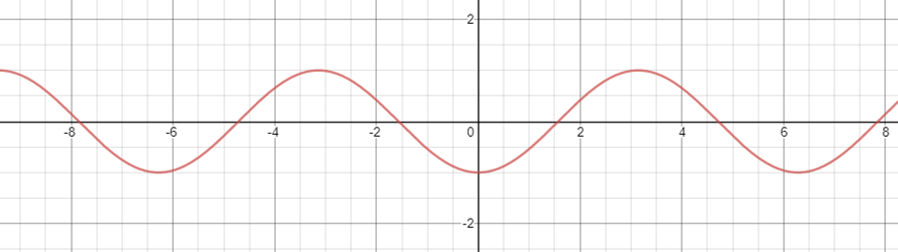Example Question #4 : Phase Shifts

Consider the function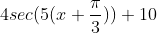.  What is the phase shift of this function?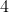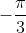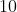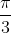Explanation:

The general form for the secant transformation equation is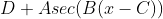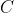represents the phase shift of the function.  When consideringwe see that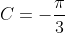.  So our phase shift isand we would shift this functionunits to the left of the original secant function’s graph.

Example Question #5 : Phase Shifts

True or False: If the function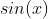has a phase shift of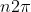, then the graph will not be changed.

True

False

True

Explanation:

This is true because the graphhas a period of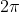, meaning it repeats itself everyunits.  So ifhas a phase shift of any multiple of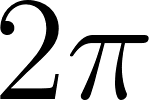, then it will just overlay the original graph.  This is shown below.  In orange is the graph ofand in purple is the graph of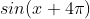.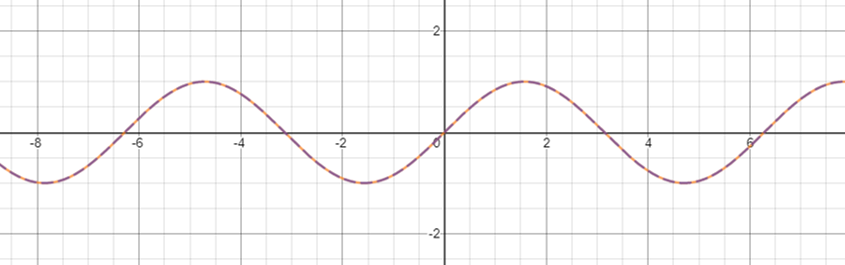Example Question #6 : Phase Shifts

Which of the following is the graph of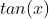with a phase shift of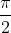?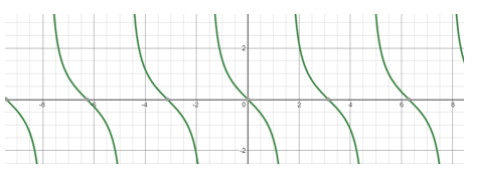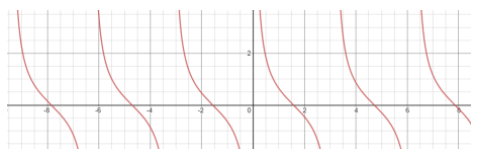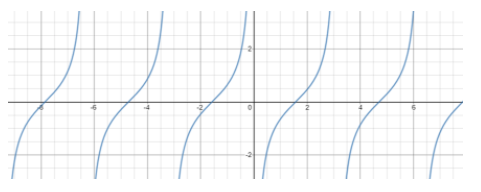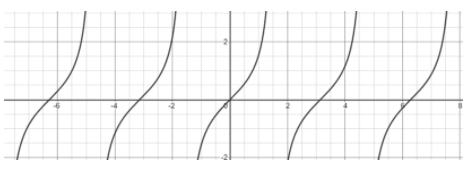Explanation:

Start this problem by graphing the function of tangent.Now we need to shift this graphto the right.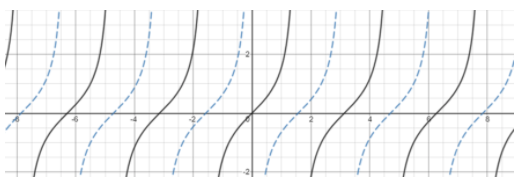Example Question #7 : Phase Shifts

True or False: The function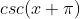has a phase shift of.

False

True

False

Explanation:

The form of the general cosecant function is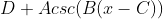.  So if we havethen, which represents the phase shift, is equal to.  This gives us a phase shift of.

Example Question #8 : Phase Shifts

Which of the following is the phase shift of the function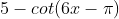?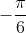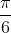Explanation:

The general form of the cotangent function is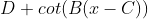.  So first we need to get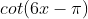into the form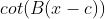.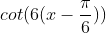From this we see that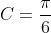giving us our answer.